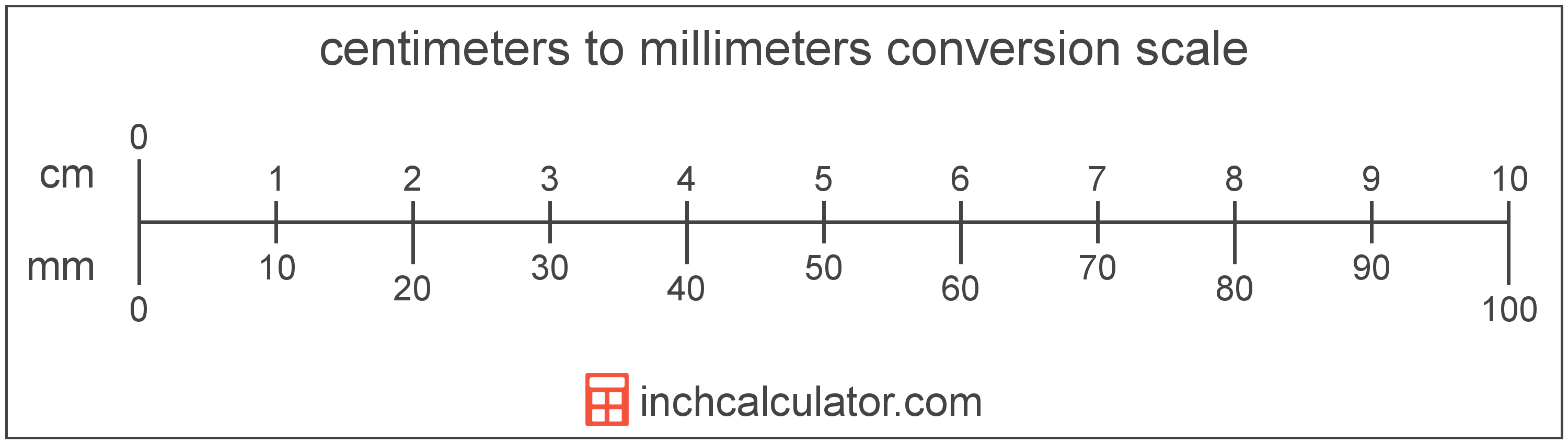# Centimeters to Millimeters Converter

Enter the length in centimeters below to get the value converted to millimeters.

Results in Millimeters:1 cm = 10 mm

Do you want to convert millimeters to centimeters?

## How to Convert Centimeters to Millimeters

To convert a measurement in centimeters to a measurement in millimeters, multiply the length by the following conversion ratio: 10 millimeters/centimeter.

Since one centimeter is equal to 10 millimeters, you can use this simple formula to convert:

millimeters = centimeters × 10

The length in millimeters is equal to the length in centimeters multiplied by 10.

For example, here's how to convert 5 centimeters to millimeters using the formula above.
millimeters = (5 cm × 10) = 50 mm### How Many Millimeters Are in a Centimeter?

There are 10 millimeters in a centimeter, which is why we use this value in the formula above.

1 cm = 10 mm

Our inch fraction calculator can add centimeters and millimeters together, and it also automatically converts the results to US customary, imperial, and SI metric values.

## What Is a Centimeter?

One centimeter is equal to one-hundredth (1/100) of a meter, which is defined as the distance light travels in a vacuum in 1/299,792,458 of a second. One centimeter is equal to 0.393701 inches.

The centimeter, or centimetre, is a multiple of the meter, which is the SI base unit for length. In the metric system, "centi" is the prefix for hundredths, or 10-2. Centimeters can be abbreviated as cm; for example, 1 centimeter can be written as 1 cm.

Metric rulers typically have 30 cm, which are represented by 30 large tick marks. To get a rough idea of the actual length of a centimeter, a standard number 2 pencil is just about 1 cm thick.

## What Is a Millimeter?

One millimeter is equal to one-thousandth (1/1,000) of a meter, which is defined as the distance light travels in a vacuum in 1/299,792,458 of a second. One millimeter is equal to 0.0393701 inches.

The millimeter, or millimetre, is a multiple of the meter, which is the SI base unit for length. In the metric system, "milli" is the prefix for thousandths, or 10-3. Millimeters can be abbreviated as mm; for example, 1 millimeter can be written as 1 mm.

Millimeters are often represented by the smallest ticks on most metric rulers. To get a reference point of the size, the thickness of a US dime is 1.35mm.

We recommend using a ruler or tape measure for measuring length, which can be found at a local retailer or home center. Rulers are available in imperial, metric, or a combination of both values, so make sure you get the correct type for your needs.

Need a ruler? Try our free downloadable and printable rulers, which include both imperial and metric measurements.

## Centimeter to Millimeter Conversion Table

Table showing various centimeter measurements converted to millimeters.
Centimeters Millimeters
0.1 cm 1 mm
0.2 cm 2 mm
0.3 cm 3 mm
0.4 cm 4 mm
0.5 cm 5 mm
0.6 cm 6 mm
0.7 cm 7 mm
0.8 cm 8 mm
0.9 cm 9 mm
1 cm 10 mm

## References

1. United States Mint, Coin Specifications, https://www.usmint.gov/learn/coin-and-medal-programs/coin-specifications